# JP2005286437A - Creation of digest in image group - Google Patents

## Info

Publication number
JP2005286437A
JP2005286437A JP2004093916A JP2004093916A JP2005286437A JP 2005286437 A JP2005286437 A JP 2005286437A JP 2004093916 A JP2004093916 A JP 2004093916A JP 2004093916 A JP2004093916 A JP 2004093916A JP 2005286437 A JP2005286437 A JP 2005286437A
Authority
JP
Japan
Prior art keywords
image
thinning
value
rank
index value
Prior art date
Legal status (The legal status is an assumption and is not a legal conclusion. Google has not performed a legal analysis and makes no representation as to the accuracy of the status listed.)
Pending
Application number
JP2004093916A
Other languages
Japanese (ja)
Inventor
Tatsuya Hosoda
Junichiro Shinozaki

Original Assignee
Seiko Epson Corp
セイコーエプソン株式会社
Priority date (The priority date is an assumption and is not a legal conclusion. Google has not performed a legal analysis and makes no representation as to the accuracy of the date listed.)
Filing date
Publication date
Application filed by Seiko Epson Corp, セイコーエプソン株式会社 filed Critical Seiko Epson Corp
Priority to JP2004093916A priority Critical patent/JP2005286437A/en
Publication of JP2005286437A publication Critical patent/JP2005286437A/en
Application status is Pending legal-status Critical

• 238000000034 methods Methods 0 abstract 1

## Images

•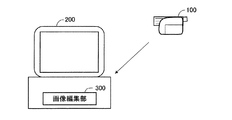•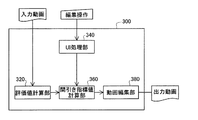••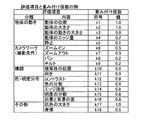•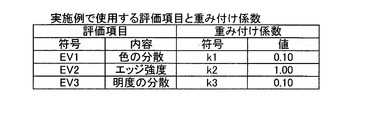•••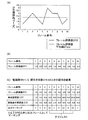••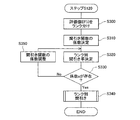•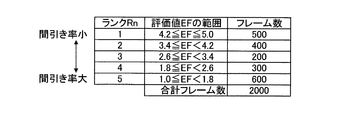••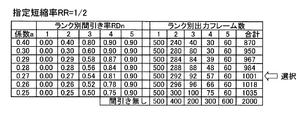•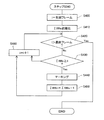•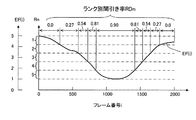•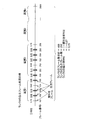•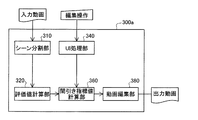•## Abstract

PROBLEM TO BE SOLVED: To provide a technique capable of automatically creating a digest using an index other than voice.
For each image in an image group composed of a plurality of images arranged in a predetermined order, an evaluation value relating to the image characteristics of each image is calculated. Then, a thinning index value associated with the thinning rate of the image at the time of creating the digest is calculated based on the evaluation value. Then, in accordance with the thinning index value, it is determined whether or not each image in the image group is thinned, and a digest composed of images that have not been thinned is created.
[Selection] Figure 8

## Description

The present invention relates to a technique for creating a digest of an image group by thinning out some images of the image group arranged in a predetermined order.

As a technique for creating a digest of a moving image, those described in the following patent documents are known. In the technique described in Patent Document 1, a moving image is shortened according to the sound data of the moving image.

JP 2002-309786 A JP 2002-142189 A

However, the conventional method has a problem that even if it is important as an image, there is a frequent occurrence of being excluded from the digest because there is no sound.

An object of the present invention is to provide a technique capable of automatically creating a digest using an index other than voice.

In order to achieve the above object, an image processing apparatus according to the present invention creates a digest of a group of images by thinning out a part of an image group composed of a plurality of images arranged in a predetermined order. An image processing apparatus for performing
With respect to each image of the image group, an evaluation value calculation unit that calculates an evaluation value related to image characteristics of each image;
A decimation index value calculation unit that calculates a decimation index value associated with an image decimation rate at the time of creating a digest based on the evaluation value;
Determining whether to thin out each image in the image group according to the thinning index value, and creating a digest composed of images that have not been thinned;
It is characterized by providing.

According to this image processing apparatus, the thinning index value is calculated using the evaluation value related to the image characteristics of the image, and it is determined whether or not each image is thinned based on the thinning index value. Can be used to automatically create a digest.

The evaluation value preferably includes a value obtained by analyzing a pixel value of each image.

According to this configuration, it is possible to more correctly determine whether to thin out each image.

The evaluation value is preferably a value obtained by integrating individual evaluation values related to a plurality of predetermined evaluation items.

According to this configuration, since the evaluation value is determined according to more various evaluation items, it is possible to more correctly determine whether to thin out each image.

The thinning index value calculation unit may determine the thinning index value so as to achieve the shortening rate when receiving the designation for the digest shortening rate for the image group.

The thinning index value calculation unit calculates an average value of the evaluation values through the image group, and sets a value obtained by dividing the average value of the evaluation values by the shortening rate as the thinning index value,
The digest creation unit
(I) a process of selecting the images in the image group one by one according to the predetermined order;
(Ii) a process of accumulating the evaluation value relating to the selected image;
(Iii) a process of thinning out the selected image when the accumulated evaluation value exceeds the thinning index value;
May be repeatedly executed.

According to this configuration, it is possible to easily determine whether or not each image is thinned out according to the relationship between the thinning index value and the accumulated value of the evaluation values of each image.

The thinning index value calculation unit may classify the entire range of the evaluation values through the image group into a plurality of ranks, and set the thinning index value for each rank.

According to this configuration, since the thinned evaluation value is set according to the range of the evaluation value, it is possible to prevent the thinning index value from being set excessively finely, and as a result, the thinning rate changes frequently. Can be prevented.

The thinning index value calculation unit
Decide the thinning rate by rank according to the range of evaluation values of each rank,
Determining a reduction ratio by rank obtained by subtracting the thinning ratio by rank from 1 as the thinning index value for each rank;
For each rank,
(I) a process of selecting images belonging to the rank one by one according to a predetermined order;
(Ii) a process of accumulating the rank-specific reduction ratio every time one image is selected;
(Iii) a process of thinning out the selected image when the accumulated reduction ratio by rank exceeds a predetermined threshold;
May be repeatedly executed.

According to this configuration, it is possible to easily determine whether or not to thin out each image according to the reduction ratio for each rank.

The image group is a frame image group constituting a moving image,
The evaluation value calculation unit calculates a scene evaluation value representing each scene section from evaluation values of a plurality of frame images in each scene section when the moving image is divided into a plurality of scene sections.
The decimation index value calculation unit may calculate one decimation index value indicating a decimation rate applied throughout the same scene segment for each scene segment in accordance with the scene evaluation value. .

According to this configuration, since the thinning rate is constant for each scene section, it is possible to prevent the thinning rate from changing frequently.

The present invention can be realized in various forms. For example, an image processing method and apparatus, a moving image editing method and apparatus, a computer program for realizing the functions of the method or apparatus, and the computer program Can be realized in the form of, for example, a recording medium on which data is recorded, a data signal including the computer program and embodied in a carrier wave.

Next, embodiments of the present invention will be described in the following order.
A. First embodiment:
B. Second embodiment:
C. Third embodiment:
D. Variation:

A. First embodiment:
FIG. 1 is an explanatory diagram showing an image processing system as an embodiment of the present invention. This image processing system includes a digital video camera 100 and a computer 200. The computer 200 includes an image editing unit 300 that executes a moving image editing process. The image editing unit 300 can perform various editing processes such as creating a digest for a moving image shot by the video camera 100.

FIG. 2 is a block diagram illustrating an internal configuration of the image editing unit 300. The image editing unit 300 includes an evaluation value calculation unit 320, a UI processing unit 340, a thinning index value calculation unit 360, and a moving image editing unit 380. The functions of these parts will be described later. The functions of these units are realized by the computer 200 executing a computer program using a CPU (processor) and a memory (not shown).

FIG. 3 is a flowchart illustrating a processing procedure in the embodiment. In step S100, the user selects a moving image to be processed (also referred to as “input moving image”), instructs the creation of the digest, and specifies a shortening rate. A moving image is composed of a plurality of frame images. Hereinafter, the frame image is also simply referred to as “frame”. The “shortening rate” means the digest length relative to the original video length. For example, when the digest length is 1/10 of the original moving image, the shortening rate is 1/10. Note that a default value (for example, 1/10) can be used as the shortening rate.

In step S110, the evaluation value calculation unit 320 calculates an evaluation value for each frame in the moving image. FIG. 4 shows an example of evaluation items to be evaluated value calculation and weighting coefficients. As in this example, many evaluation items can be used, but in this embodiment, three evaluation items of “color dispersion”, “edge strength”, and “lightness dispersion” shown in FIG. An example using only the following will be described. The evaluation values related to these three evaluation items are calculated as follows, for example.

(1) Calculation of frame evaluation value EV1 related to color dispersion:
The frame evaluation value EV1 related to the color dispersion is set to be higher as the color dispersion in the frame image is larger. When calculating the evaluation value EV1, first, a histogram is created for each color component related to the hue for all pixels in each frame image. For example, when the frame image is expressed in the RGB color system, histograms are created for three components R, G, and B. In addition, when the frame image is expressed in the YCrCb color system, histograms are calculated for the color difference components Cr and Cb. Then, a statistical variance for each color component is calculated from these histograms. The frame evaluation value EV1 related to the color dispersion is set to be higher as the dispersion is larger. FIG. 6A shows an example of the relationship between the color dispersion and the evaluation value EV1. In this example, the evaluation value EV1 increases linearly from 0 until the variance of the color component reaches a predetermined value V1, and when the variance of the color component is equal to or greater than this value V1, the predetermined maximum value EV1max is maintained. The The reason why the maximum value EV1max is set is to prevent the frame evaluation value EV1 of color dispersion from becoming excessive compared to other evaluation values. However, the maximum value EV1max may not be provided. FIG. 6B shows another example of the relationship between the color dispersion and the evaluation value EV1. In this example, the evaluation value EV1 is kept at 0 until the variance of the color components reaches a predetermined value V0. When the variance of the color components is between the values V0 and V1, the evaluation value EV1 is from 0 to the maximum value EV1max. Increases linearly. If the evaluation value EV1 related to color dispersion is set so as to increase as the color dispersion in the frame image increases as in the examples of FIGS. 6A and 6B, the color in the frame image It is possible to obtain an evaluation value that objectively indicates the distribution state of.

(2) Calculation of frame evaluation value EV2 related to edge strength:
The frame evaluation value EV2 related to the edge strength is set so as to increase as the number of stronger edges increases in the frame image. When calculating the evaluation value EV2, first, the edge strength is calculated for all the pixels in each frame image. As the edge strength, for example, an absolute value obtained as a result of applying a Laplacian filter to the luminance value of each pixel can be employed. When the frame image is expressed in the RGB color system, the edge strength is calculated using the G component. Further, when the frame image is expressed in the YCrCb color system, the edge strength is calculated using the luminance component Y. Next, an edge strength point that increases as the edge strength of each pixel increases is calculated for each pixel. For example, the edge strength itself may be used as the edge strength point, or the edge strength point may be calculated as a function of the edge strength. Then, the total value of the edge strength points in the frame image is calculated according to the following equation (1).

In this equation, the edge intensity point E (x, y) is an absolute value as a result of applying a Laplacian filter to the pixel value at the pixel position (x, y) in the frame image. The coefficient C is a weighting coefficient determined according to the edge strength E (x, y) at the position (x, y). The coefficient C is preferably set so as to increase as the edge strength increases. For example, the coefficient C can be calculated using a linear increase function or a logarithmic function. Alternatively, C = 1 may be constant. Then, the frame evaluation value EV2 related to the edge strength is calculated according to the edge strength point total value. FIG. 6C shows an example of the relationship between the edge strength point total value and the evaluation value EV2. In this example, the evaluation value EV2 is kept at 0 until the edge strength point total value reaches a predetermined value G0, the edge strength point total value is between the values G0 and G1, and the evaluation value EV2 is 0 to the maximum value. It increases linearly to EV2max. However, a graph similar to that shown in FIG. As described above, when the frame evaluation value EV2 related to the edge strength is set so as to increase as the total value of the edge strengths in the frame image increases, an evaluation value that objectively indicates the edge strength in the frame image is obtained. be able to.

(3) Calculation of frame evaluation value EV3 related to brightness variance:
The frame evaluation value EV3 related to the variance in brightness is set to be higher as the various brightness levels from bright pixels to dark pixels exist in the frame image. In this embodiment, the luminance value Y of the YCbCr color system is used for the calculation of the evaluation value EV3. First, a histogram of luminance values is created for all pixels in each frame image. Then, from this histogram, a statistical variance for the luminance value Y is calculated. This dispersion is called “luminance dispersion” or “lightness dispersion”. The frame evaluation value EV3 related to brightness is set so as to increase as the brightness variance increases. The relationship between the brightness variance and the evaluation value EV3 can be set similarly to the relationship between the color variance and the evaluation value EV1 (for example, FIGS. 6A and 6B).

Note that, when calculating the above-described histogram and edge strength, instead of using all the pixels in each frame image, only a plurality of pixels selected at a certain sampling interval may be used. When creating a histogram, values may be divided into a plurality of ranges, and pixels may be grouped in the ranges, and the frequency may be obtained for each group. For example, when the red component R can take a value of 0 to 255, this range is divided into 16 groups of 0 to 5, 6 to 9, 10 to 15, 240 to 255, and each group is divided into each group. You may obtain | require the total value of the number of pixels which belong. At this time, a group having a smaller number of pixels than the threshold can be excluded from the population at the time of variance calculation.

In step S110 of FIG. 3, the frame total evaluation value EF (i) of each frame image is further calculated according to the following equation (2).

Here, EVn is an evaluation value of the nth evaluation item, kn is a weighting coefficient for the nth evaluation item, and p is the number of evaluation items. The weighting coefficient kn of each evaluation item can be set to an arbitrary value. In the example of FIG. 5, k1 = k3 = 0.1 and k2 = 1.0. That is, the edge strength has the highest weight among the three evaluation items.

The overall frame evaluation value EF (i) can be calculated using an evaluation value related to at least one evaluation item. However, if a comprehensive evaluation value corresponding to each weight is used for the evaluation values for a plurality of evaluation items, there is a possibility that the frame image to be thinned out and the frame image to be left out can be more appropriately determined when the digest is created. There is an advantage of high.

The evaluation items preferably include those relating to the image characteristics of the frame image. This is because, for example, it is more likely that the frame image to be thinned out can be determined more correctly than when only evaluation items that are not image characteristics such as speech are used. Note that the evaluation value related to the image characteristics of the frame image is not directly related to the pixel value of the frame image, such as shooting conditions, and is obtained by analyzing the pixel value of the frame image, such as contrast. Exists. Among these, if an evaluation value obtained by analyzing a pixel value is used, an evaluation value that more accurately reflects the actual image characteristics can be obtained.

In the first embodiment, as will be described later, whether or not to thin out each frame image at the time of creating a digest is determined based on the overall frame evaluation value EF (i) of each frame image. Therefore, hereinafter, “frame comprehensive evaluation value EF (i)” is also simply referred to as “frame evaluation value EF”.

When the frame evaluation value EF (i) is calculated in this way, in step S120 in FIG. 3, the thinning index value calculation unit 360 and the moving image editing unit 380 cooperate to execute the thinning process.

FIG. 7 is a flowchart showing the detailed procedure of step S120. In step S200, the thinning index value calculation unit 360 calculates the average value EFave of the frame evaluation values EF (i) over the entire moving picture by the following equation (3).

Here, F is the number of frame images in the moving image.

FIG. 8A shows an example of a change in the frame evaluation value EF (i) in the moving image. Here, for the sake of simplicity, it is assumed that the entire moving image is composed of 10 frames. The frame evaluation value average value EFave is 2.2.

In step S210, the decimation index value calculation unit 360 calculates a decimation coefficient D as a decimation index value by the following equation (4).

Here, RR is the shortening rate specified in step S100.

As will be described later, the thinning coefficient D is a value used as a threshold value when determining whether to thin out each frame image. When the frame evaluation value average value EFave is 2.2 and the shortening rate RR is 1/3, the thinning coefficient D is 6.6 as given by the following equation.

The processing after step S220 in FIG. 7 is executed by the moving image editing unit 380. First, in step S220, the initial frame number of the moving image is set as the initial value setting of the current frame number i. The “current frame number” means a number indicating the frame of interest. In the example of FIG. 8, the initial value of the current frame number i is 1.

In step S230, the accumulated value ΣEF of the frame evaluation value EF is initialized to zero. In step S240, the frame evaluation value EF (i) for the frame with the current frame number i is added to the current accumulated value ΣEF to update the accumulated value ΣEF.

In step S250, the accumulated value ΣEF and the thinning coefficient D are compared. When the accumulated value ΣEF is greater than or equal to the thinning coefficient D, the i-th frame is selected as the output frame (step S260). Here, the “output frame” means a frame image used for the digest. On the other hand, when the accumulated value ΣEF is less than the thinning coefficient D, it is determined that the i-th frame is not set as an output frame (step S270). Whether to make an output frame is stored by marking or flagging each frame.

If the current frame is selected as the output frame in step S260, the accumulated value ΣEF is updated by subtracting the thinning coefficient D from the accumulated value ΣEF in the next step S280. Then, the process of steps S240 to S280 is repeatedly executed while incrementing the current frame number i until the last frame image of the moving image is reached (steps S290 and S295).

FIG. 8C shows a thinning result when the shortening rate RR is 1/3 and the thinning coefficient D is 6.6. Here, since the fourth frame is an output target, the accumulated value ΣEF in the fifth frame is a value smaller by 6.6 (= decimation coefficient D) than the simple accumulated value (= 9). become. Similarly, the seventh and ninth frames are also selected as output targets. As a result, only 3 frames out of 10 frames are selected as output targets. The actual shortening rate at this time is 3/10, and the shortening rate RR = 1/3 designated in step S100 is almost realized.

FIG. 9A shows a thinning result when the shortening rate RR is 1/2 and the thinning coefficient D is 4.4. The actual shortening rate at this time is 4/10, and it can be understood that the designated shortening rate RR = 1/2 is almost realized. FIG. 9B shows a thinning result when the shortening rate RR is 4/5 and the thinning coefficient D is 2.75. The actual shortening rate at this time is 8/10, and it can be understood that the designated shortening rate RR = 4/5 is realized.

As can be understood from these examples, when the accumulated value ΣEF of the frame evaluation value is greater than or equal to the thinning coefficient D, the current frame is selected as the output frame and this value D is subtracted from the accumulated value ΣEF, The specified shortening rate RR can be achieved by not selecting the current frame as an output frame when the accumulated value ΣEF of the current frame is less than the thinning coefficient D.

In step S <b> 130 of FIG. 3, the moving image editing unit 380 creates a digest (decimated moving image) using the frame selected as the output target. In the present embodiment, a thinning coefficient D is determined so as to achieve a desired shortening rate RR specified by the user, and by using this thinning coefficient D, it is determined whether or not each frame is thinned, and thereby a desired reduction rate RR is determined. It is possible to easily create a digest having a shortening rate RR. In particular, whether or not to perform thinning out for each frame is determined according to the evaluation value EF (i) of each frame, so that more important frames are included and less important frames are not included. It is possible to create a simple digest.

B. Second embodiment:
In the second embodiment, the apparatus configuration is the same as that of the first embodiment (FIGS. 1 and 2), and the entire processing procedure is the same as that of the first embodiment (FIG. 3). The procedure is different from that of the first embodiment (FIG. 7).

FIG. 10 is a flowchart showing a detailed procedure of step S120 in the second embodiment. In step S300, the thinning index value calculation unit 360 performs rank classification (grouping) of the frame evaluation values EF (i). In this embodiment, rank 1, rank 2,... And a plurality of ranks Rn are set in order from the highest evaluation value EF (i). This ranking can be realized, for example, by dividing equally between the maximum value EFmax and the minimum value EFmin of the frame evaluation value EF (i) in the moving image. At this time, the range of the frame evaluation value EF (i) belonging to the nth rank Rn is given by the following equation (6).

Here, Rnum is the number of ranks.

FIG. 11 shows an example in which the maximum evaluation value EFmax is 5, the minimum evaluation value EFmin is 1, and the rank number Rnum is 5. The number of frames belonging to each rank is illustrated in the rightmost column. In this example, it is assumed that the entire moving image is composed of 2000 frames.

In step S310, the decimation index value calculation unit 360 sets a decimation function RDn (Rn) for determining the decimation rate RDn for each rank Rn. As the thinning function RDn (Rn), for example, the following equation (7) can be used.

Here, a, b, and c are coefficients.

The thinning rate RDn on the left side of the equation (7) is a value obtained by subtracting the shortening rate RR from 1 (= 1−RR). As described above, the shortening rate RR is the ratio of the length of the digest to the original moving image. Therefore, the thinning rate RDn corresponds to a value obtained by dividing the number of frames deleted at the time of thinning by the number of frames of the entire moving image. For example, when the thinning rate RDn is 0.1, frames are thinned out at a rate of 1 out of 10 frames, and 9 out of 10 frames remain.

The coefficients a, b, and c of the RDn (Rn) of the thinning function are set so that the thinning rate RDn decreases as the rank increases. Here, “high rank” means a rank having a high evaluation value EF. For example, among the five ranks 1 to 5 in FIG. 11, the thinning rate RD1 of the highest rank 1 is set to a value smaller than the thinning rate RD5 of the lowest rank 5.

Of the three coefficients a, b, and c, two coefficients b and c are set to preferred values in step S310. For example, when b = 0 and c = 1, the thinning rate RD1 of the highest rank 1 can be set to 0 (no thinning). In general, as the coefficient c is increased, the thinning rate RDn at the higher rank tends to decrease. Further, the overall thinning rate tends to increase as the coefficient b increases. However, as shown in the above equation (7), it is preferable to limit the thinning rate RDn of each rank between the lower limit value RDmin and the upper limit value RDmax. This is because if such a restriction is not provided, when the coefficient a is changed, the calculation result of the thinning-out rate RDn may be negative or larger than 1. When the upper limit value RDmax of the thinning rate is increased, the thinning rate RDn is increased at a low rank. Further, when the lower limit value RDmin is reduced, the thinning rate at a high rank is reduced. The lower limit value RDmin and the upper limit value RDmax are set to arbitrary values in the range of 0 to 1.

In the second embodiment, the coefficients b and c, the lower limit value RDmin and the upper limit value RDmax in the RDn (Rn) of the thinning function of the equation (7) are set to predetermined values, respectively, and the coefficient a is changed. Further, the rank-specific thinning rate RDn can be adjusted. FIG. 12 shows an example of the rank-specific thinning rate RDn obtained by adjusting the coefficient a when b = 0, c = 1, RDmin = 0, and RDmax = 0.9. In the example of FIG. 12, the rank-specific thinning rate RDn is indicated by a continuous broken line, but only meaningful values are circled.

As can be understood from FIG. 12, the coefficient a is a value indicating the slope of change in the thinning rate RDn between adjacent ranks. Therefore, by adjusting the coefficient a, the rank-specific thinning rate RDn can be changed, and as a result, the digest length can be adjusted to a desired value.

Note that four values, b, c, RDmin, and RDmax, are determined in step S310 in FIG. As these values, values selected in advance may be used, or values designated by the user may be used.

In step S320, the decimation index value calculation unit 360 adjusts the coefficient a to determine the decimation rate RDn for each rank so that the length of the entire digest achieves the specified shortening rate RR. Note that the adjustment interval of the coefficient a is preferably determined in advance. For example, the value of the coefficient a is gradually increased from a = 0 to a constant width (for example, 0.01), and the total number of frames after thinning is calculated from the reduction rate RR specified by the user. Search for the value of the coefficient a that matches. Then, the rank-specific thinning rate RDn obtained by using the value of the coefficient a is adopted as the thinning rate used in the actual thinning process.

FIG. 13 shows an example of the value of the coefficient a, the rank-specific thinning rate RDn, and the number of rank-specific output frames. As can be understood from this, when a = 0.27, the total number of output frames by rank is 1001 frames, which is almost ½ of the number of frames of the input moving image 2000. Therefore, the specified shortening rate RR can be achieved by using the rank-specific thinning rate RDn when a = 0.27. In determining the coefficient a, it is preferable to set in advance an allowable error of the total number of output frames.

Even if the coefficient a is adjusted in step S320, the specified reduction rate RR may not be achieved. In this case, after adjusting at least one of the other set values c, b, RDmin, and RDmax in step S350, the process returns to step S320, and the adjustment of the coefficient a and the rank-specific thinning rate RDn is performed again. In step S350, instead of adjusting the set values c, b, RDmin, and RDmax, the rank number Rnum may be increased. The upper limit of the rank number Rnum is preferably set to a value (for example, 10 times EFmax) corresponding to the maximum frame evaluation value EFmax.

Thus, when the rank-specific thinning rate RDn that achieves the specified shortening rate RR is obtained, the moving image editing unit 380 executes the thinning process in step S340.

FIG. 14 is a flowchart showing a detailed procedure of step S340. In step S400, the number of the first frame of the moving image is set as the initial value setting for the current frame number i. In step S410, the rank-specific shortening rate accumulation value ΣRRn is initialized. Here, the rank reduction rate RRn is equal to a value obtained by subtracting the rank reduction rate RDn from 1 (= 1−RRn). For example, when the rank number Rnum is 5, all the rank-specific shortening rates ΣRR1 to ΣRR5 are initialized to 0. As described above, the shortening rate RR is a value obtained by dividing the length of the digest by the length of the original moving image. The thinning rate RDn is a value obtained by dividing the number of frames deleted at the time of thinning by the original number of frames.

In step S420, it is determined whether or not the current frame number i exceeds the final frame number of the moving image. If it exceeds the final frame number of the moving image, the process of FIG. 14 is terminated, and if not, the process proceeds to step S430.

In step S430, it is determined whether the shortening rate accumulated value ΣRRn of the rank to which the current frame i belongs is 1 or more. When the shortening rate cumulative value ΣRRn is less than 1, the current frame number i is incremented in step S460, and then the process returns to step S420. On the other hand, when the shortening rate cumulative value ΣRRn is 1 or more, the current frame i is marked as an output frame (step S440). In step S450, 1 is subtracted from the shortening rate cumulative value ΣRRn of the rank to which the current frame i belongs, and then the process proceeds to step S460.

According to the processing of steps S420 to S460, as in the processing of steps S240 to S290 of FIG. 7, the desired reduction rate RRn is achieved as a whole while determining whether or not each frame image is individually set as an output frame. can do.

FIG. 15 shows an example of the change of the frame evaluation value EF (i) in the moving image, the rank Rn classification, and the rank-specific thinning rate RDn in the second embodiment. The frame evaluation value EF (i) often shows a fairly smooth change as in this example. Each frame is classified into one of five ranks Rn according to the frame evaluation value EF (i), and a thinning rate RDn according to this rank Rn is assigned to each frame. Therefore, the thinning rate RDn is 0, 0 (no thinning) in the first part of the moving image, and then gradually changes in a stepped manner such as 0.27, 0.54.

FIG. 16 shows a state in which the frame image belonging to rank 2 is thinned out according to the flow of FIG. This example relates to a moving image different from that in FIG. 15, and m frame images belonging to rank 2 include a section 1 with a frame number i of 101 to 105 and a section 2 with a frame number i of 200 to 206. It is divided into sections. Frames belonging to other ranks are irrelevant for the thinning-out process of frames belonging to rank 2. The state of FIG. 16 can be considered to be a state in which frames of the same rank in the input moving image are virtually arranged in time series. During the thinning process, each time a frame belonging to rank 2 appears, a certain rank-specific shortening rate RR2 is sequentially added to update the accumulated value ΣRR2. When the accumulated value ΣRR2 becomes 1 or more, the frame is selected as an output frame, and 1 is subtracted from the accumulated value ΣRR2.

14 and 16, when the thinning-out rate RDn is larger than 0, the shortening rate accumulated value ΣRRn of the first frame never becomes 1 or more, so the first frame is not an output target. . In order to avoid such a situation, the top frame may be always selected as the output frame. The same applies to the first embodiment described above.

As described above, in the second embodiment, the frame evaluation value EF (i) is divided into a plurality of ranks, and a constant thinning rate RDn (that is, a shortening rate RRn) that is different for each rank is set. Is created at a specified reduction rate RR. Therefore, as in the first embodiment, the change in the thinning rate can be made smoother than in the case where each frame evaluation value EF (i) is directly used to determine whether to thin out each frame image. it can. As a result, there is an advantage that a more natural digest can be obtained.

C. Third embodiment:
FIG. 17 is a block diagram showing the internal configuration of the image editing unit 300a in the third embodiment. The image editing unit 300a is obtained by adding a scene dividing unit 310 to the image editing unit 300 of the first embodiment shown in FIG. 2, and the other configuration is the same as that of the first embodiment.

The scene division unit 310 divides the moving image to be processed into a plurality of divided scenes. Here, the “divided scene” means one continuous period in the moving image, and is hereinafter also referred to as “scene section” or simply “scene”. The scene can be divided by various methods. For example, the scene may be divided according to the length of time. Alternatively, it is also possible to set a boundary where the index value relating to a predetermined item (brightness or color histogram, luminance or color difference between frames, sound, etc.) greatly changes as the boundary of the divided scene.

FIG. 18 is an explanatory diagram illustrating an example of a change in the frame evaluation value EF (i) in the moving image according to the third embodiment. The change of the frame evaluation value EF (i) itself is the same as that shown in FIG. In this example, the entire moving image is divided into four divided scenes every 500 frames. The evaluation value calculation unit 320 calculates an average value ES (j) of the frame evaluation values EF (i) for each divided scene. Here, j is the number of the divided scene. This average value ES is referred to as “scene evaluation value ES”.

In the third embodiment, during the thinning process in step S120 (FIG. 3), the frame evaluation values EF (i) of all the frames in each divided scene are equal to the scene evaluation values ES (j) of the divided scenes. The thinning process is executed assuming that As the thinning-out method, either the method of the first embodiment (FIG. 7) or the method of the second embodiment (FIG. 10) described above may be used. That is, in the method of FIG. 7, the frame evaluation value EF (i) of each frame is regarded as being equal to the scene evaluation value ES (j) of the divided scene to which the frame belongs, and the thinning process is executed. Further, in the method of FIG. 10, the rank reduction rate RDn for each frame is determined according to the scene evaluation value ES (j) of the divided scene to which the frame belongs, and the thinning process is performed according to the rank reduction rate RDn. Executed.

As described above, in the third embodiment, since the thinning process is executed assuming that all the frames in each divided scene have the same frame evaluation value (= ES), the thinning rate RD ( That is, the shortening rate RR) is kept constant. Therefore, it is possible to create a natural digest.

D. Variation:
The present invention is not limited to the above-described examples and embodiments, and can be implemented in various modes without departing from the gist thereof. For example, the following modifications are possible.

D1. Modification 1:
In the above embodiment, the thinning coefficient D and the rank-specific shortening rate RRn are used as the thinning index value indicating whether or not each frame image is thinned, but other thinning index values may be used. In general, as the decimation index value, an index value associated with an image decimation rate at the time of digest creation can be used.

D2. Modification 2:
In the above embodiment, the processing is performed on the moving image supplied from the digital video camera 100 and stored in the computer 200, but various moving images can be used as the processing target moving image. For example, the present invention can be applied to a case where a digest is created from a moving image distributed by broadcasting.

The present invention can also be applied when a user requests digest creation processing from a video distribution server. At this time, the moving image itself is stored in a server connected to the computer 200 via the network. When the user issues a digest creation instruction on the computer 200, a digest of the moving image is created on the server side and distributed to the computer 200. In this case, some of the units shown in FIG. 2 (for example, the evaluation value calculation unit 320, the thinning index value calculation unit 360, and the moving image editing unit 380) may be provided on the server side.

D3. Modification 3:
In the above embodiment, the digest is created so as to achieve the pre-designated shortening rate RR. However, it is also possible to create a digest without using the shortening rate RR. For example, in the second embodiment, various setting values a, b, c, RDmin, and RDmax in equation (7) are set in advance, and the rank-specific shortening rate RDn is determined regardless of the overall shortening rate RR. It may be. In this case, since the reduction rate of the entire digest depends on the original moving image, a constant reduction rate is not achieved, but there is no practical problem.

D4. Modification 4:
In the above embodiment, a moving image is used as an image processing target. However, a still image can be processed. In this case, the present invention is applied when selecting a plurality of still images to be used for the next predetermined image processing (for example, pasting into an album or printing) from a plurality of still images. Can do.

D5. Modification 5:
In the above embodiment, a part of the configuration realized by hardware may be replaced with software, and conversely, a part of the configuration realized by software may be replaced by hardware. For example, part of the functions of the image editing unit 300 (FIG. 2) can be executed by a hardware circuit.

It is explanatory drawing which shows the image processing system as one Example of this invention. It is a block diagram which shows the internal structure of an image editing part. It is a flowchart which shows the process sequence in an Example. It is explanatory drawing which shows the example of the evaluation item used as the object of evaluation value calculation. It is explanatory drawing which shows the other example of the evaluation item used as the object of evaluation value calculation. It is a graph which shows the calculation method of an evaluation value. It is a flowchart which shows the detailed procedure of step S120 in 1st Example. Explanatory drawing which shows an example of the frame evaluation value EF (i) in a moving image, and a thinning result. It is explanatory drawing which shows the other thinning result in 1st Example. It is a flowchart which shows the detailed procedure of step S120 in 2nd Example. It is explanatory drawing which shows the example of ranking of the frame evaluation value EF. It is explanatory drawing which shows the example of the thinning-out rate RDn of each rank obtained by adjusting the coefficient a. It is explanatory drawing which shows the example of the coefficient a of the thinning function, the thinning rate by rank RDn, and the number of output frames by rank. It is a flowchart which shows the detailed procedure of step S340. It is explanatory drawing which shows the example of the change of the frame evaluation value EF (i) in the moving image in 2nd Example, the division | segmentation of rank Rn, and the thinning rate RDn according to rank. It is explanatory drawing which shows the mode of the thinning-out process regarding rank-2. It is a block diagram which shows the internal structure of the image editing part in 3rd Example. It is explanatory drawing which shows the example of the change of the frame evaluation value EF (i) in the moving image in 3rd Example, and the average evaluation value ES (j) for every division | segmentation scene.

Explanation of symbols

DESCRIPTION OF SYMBOLS 100 ... Digital video camera 200 ... Computer 300 ... Image editing part 310 ... Scene division | segmentation part 320 ... Evaluation value calculation part 340 ... UI processing part 360 ... Decimation index value calculation part 380 ... Movie editing part

## Claims (10)

1. An image processing apparatus for creating a digest of an image group by thinning out a part of an image group composed of a plurality of images arranged in a predetermined order,
With respect to each image of the image group, an evaluation value calculation unit that calculates an evaluation value related to image characteristics of each image;
A decimation index value calculation unit that calculates a decimation index value associated with an image decimation rate at the time of creating a digest based on the evaluation value;
Determining whether to thin out each image in the image group according to the thinning index value, and creating a digest composed of images that were not thinned;
An image processing apparatus comprising:
2. The image processing apparatus according to claim 1,
The image processing apparatus, wherein the evaluation value includes a value obtained by analyzing a pixel value of each image.
3. The image processing apparatus according to claim 1,
The image processing apparatus, wherein the evaluation value is a value obtained by integrating individual evaluation values related to a plurality of predetermined evaluation items.
4. The image processing apparatus according to any one of claims 1 to 3,
The thinning index value calculation unit determines the thinning index value so as to achieve the shortening rate when receiving a designation for the shortening rate of the digest for the image group.
5. The image processing apparatus according to claim 4,
The thinning index value calculation unit calculates an average value of the evaluation values through the image group, and sets a value obtained by dividing the average value of the evaluation values by the shortening rate as the thinning index value,
The digest creation unit
(I) a process of selecting the images in the image group one by one according to the predetermined order;
(Ii) a process of accumulating the evaluation value relating to the selected image;
(Iii) a process of thinning out the selected image when the accumulated evaluation value exceeds the thinning index value;
An image processing apparatus that repeatedly executes.
6. The image processing apparatus according to any one of claims 1 to 4,
The thinning index value calculation unit classifies the entire range of the evaluation values through the image group into a plurality of ranks, and sets the thinning index value for each rank.
7. The image processing apparatus according to claim 6,
The thinning index value calculation unit
Decide the thinning rate by rank according to the range of evaluation values of each rank,
Determining a reduction ratio by rank obtained by subtracting the thinning ratio by rank from 1 as the thinning index value for each rank;
For each rank,
(I) a process of selecting images belonging to the rank one by one according to a predetermined order;
(Ii) a process of accumulating the rank-specific reduction ratio every time one image is selected;
(Iii) a process of thinning out the selected image when the accumulated reduction ratio by rank exceeds a predetermined threshold;
An image processing apparatus that repeatedly executes.
8. An image processing apparatus according to any one of claims 1 to 7,
The image group is a frame image group constituting a moving image,
The evaluation value calculation unit calculates a scene evaluation value representing each scene section from evaluation values of a plurality of frame images in each scene section when the moving image is divided into a plurality of scene sections.
The thinning index value calculation unit is configured to calculate one thinning index value indicating a certain thinning rate applied through the same scene section for each scene section in accordance with the scene evaluation value.
9. An image processing method for creating a digest of an image group by thinning out a part of an image group composed of a plurality of images arranged in a predetermined order,
(A) for each image in the image group, calculating an evaluation value related to image characteristics of each image;
(B) calculating a decimation index value associated with an image decimation rate at the time of creating a digest based on the evaluation value;
(C) determining whether to thin out each image in the image group according to the thinning index value, and creating a digest composed of images that have not been thinned;
An image processing method comprising:
10. A program for creating a digest of the image group by thinning out a part of an image group composed of a plurality of images arranged in a predetermined order,
With respect to each image of the image group, an evaluation value calculation function for calculating an evaluation value related to image characteristics of each image;
A decimation index value calculation function for calculating a decimation index value associated with an image decimation rate at the time of creating a digest based on the evaluation value;
Determining whether to thin out each image in the image group according to the thinning index value, and creating a digest composed of images that are not thinned;
A program characterized by causing a computer to realize.
JP2004093916A 2004-03-29 2004-03-29 Creation of digest in image group Pending JP2005286437A (en)

## Priority Applications (1)

Application Number Priority Date Filing Date Title
JP2004093916A JP2005286437A (en) 2004-03-29 2004-03-29 Creation of digest in image group

## Applications Claiming Priority (1)

Application Number Priority Date Filing Date Title
JP2004093916A JP2005286437A (en) 2004-03-29 2004-03-29 Creation of digest in image group

## Publications (1)

Publication Number Publication Date
JP2005286437A true JP2005286437A (en) 2005-10-13

# Family

## Family Applications (1)

Application Number Title Priority Date Filing Date
JP2004093916A Pending JP2005286437A (en) 2004-03-29 2004-03-29 Creation of digest in image group

## Country Status (1)

JP (1) JP2005286437A (en)

## Cited By (2)

* Cited by examiner, † Cited by third party
Publication number Priority date Publication date Assignee Title
WO2008032739A1 (en) * 2006-09-12 2008-03-20 Panasonic Corporation Content imaging device
JP2012010319A (en) * 2010-05-28 2012-01-12 Hitachi Ltd Compression method of time series data and compression device

## Cited By (4)

* Cited by examiner, † Cited by third party
Publication number Priority date Publication date Assignee Title
WO2008032739A1 (en) * 2006-09-12 2008-03-20 Panasonic Corporation Content imaging device
US8208792B2 (en) 2006-09-12 2012-06-26 Panasonic Corporation Content shooting apparatus for generating scene representation metadata
JP5022370B2 (en) * 2006-09-12 2012-09-12 パナソニック株式会社 Content shooting device
JP2012010319A (en) * 2010-05-28 2012-01-12 Hitachi Ltd Compression method of time series data and compression device

## Similar Documents

Publication Publication Date Title
US8098953B2 (en) Image processing apparatus and image processing method for adaptively processing an image using an enhanced image and edge data
US7834915B2 (en) Image processing apparatus, imaging apparatus, imaging processing method, and computer program
US5581370A (en) Image-dependent automatic area of interest enhancement
US8290263B2 (en) Image processing apparatus
EP1800259B1 (en) Image segmentation method and system
CN1278540C (en) Reducing image noise
US7953251B1 (en) Method and apparatus for detection and correction of flash-induced eye defects within digital images using preview or other reference images
US7460168B2 (en) Image processing apparatus for generating a wide dynamic range image
US7933454B2 (en) Class-based image enhancement system
JP3992177B2 (en) Image processing apparatus, image processing method, and computer program
JP3880553B2 (en) Image processing method and apparatus
JP5530563B2 (en) High dynamic range image capture and rendering
JP2004282416A (en) Method and apparatus for processing image
EP1807805B1 (en) Method and apparatus for red-eye detection in an acquired digital image
EP1231564B1 (en) Digital local control of image properties by means of masks
US20050226526A1 (en) Image processing device and method
US8355595B2 (en) Contrast enhancement methods and apparatuses
US7013025B2 (en) Image correction apparatus
JP4410304B2 (en) Visual processing device, visual processing method, image display device, television, portable information terminal, camera, and processor
JP2006510072A (en) Method and system for detecting uniform color segments
US5504524A (en) Method and apparatus for controlling color balance of a video signal
US7606462B2 (en) Video processing device and method for producing digest video data
JP3758452B2 (en) Recording medium, image processing device, and image processing method
US9344642B2 (en) Method and apparatus for capturing a first image using a first configuration of a camera and capturing a second image using a second configuration of a camera
US7599578B2 (en) Apparatus, program, and method for image tone transformation, and electronic camera